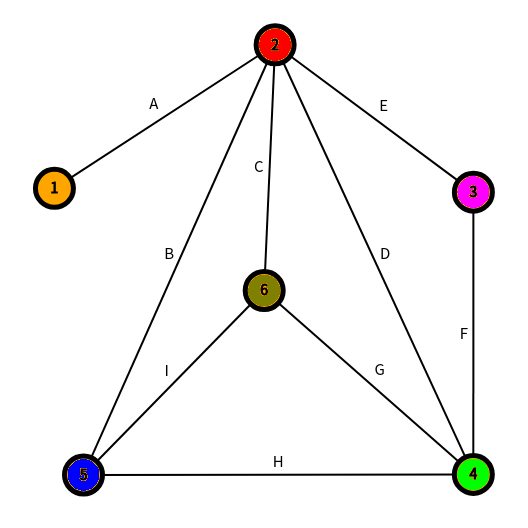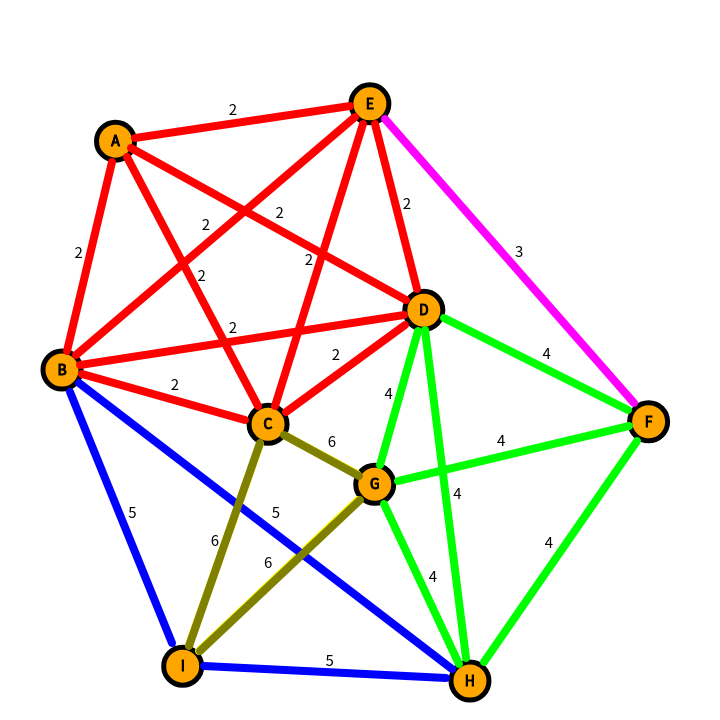# A. 串

check 失败就 break

## 40pts

``````#include<cstdio>
#include<iostream>
#include<algorithm>
#include<cstring>
#include<queue>
using namespace std;
const int N=1e5+5;
char s[N];
int ans;
int del[N];
int calc(int a,int b){// unsimilarity
int tot=0;
for(int i=0;i<b-a;i++)tot+=(s[i+a]!=s[i+b]);
}
int main(){cin>>s;
int n=strlen(s);
for(int len=2;len<n;len++){for(int i=0;i<len;i++){// 起点
int tot=1;
for(int k=0;i+k+len+len-1<n;k+=len){if(i==0)del[i+k]=calc(i+k,i+k+len);// 初始时计算 check
else del[i+k]=del[i+k-1]-(s[i+k-1]!=s[i+k+len-1])+(s[i+k+len-1]!=s[i+k+len+len-1]);//O(1) 维护 check
if(del[i+k]<=1)++tot;
else ans+=tot*(tot-1)/2,tot=1;
}
ans+=tot*(tot-1)/2;// 累加答案
}
}
cout<<ans;
return 0;
}
``````

# C. 图

## 线图

• 它的点集为 ，每个点唯一对应着原图的一条边。
• 线图上的两个点之间有边当且仅当这两个点对应的边在原图上有公共点 (注意不会有自环)。

## k=1## k=2

• 对于 中的点，其本质等价于 中的边，等价于 中两个具有公共顶点的边
• 对于 中的边，其本质等价于 中两个具有公共顶点的边，而这也等价于 中三个连续边链• 对于选择的第一条边，它对应 中的一个团，假设这条边两个端点的分别为 ，那么这个团的顶点数显然为 。显然初始时这个团有 个连通块，那么我们贪心地连接其中的任意 条边，使其连通成一个整体。这时，这个团显然可以缩点。那么在 上的缩点对应在 上的什么操作呢？——删边！既然这个团缩了点，那么其所有的边都消失了，这个团也消失了（这里的团偏指边集），不就相当于删边吗！因此，我们在原图中把这条边删除（其实就是两个点的度 -1）
• 对应之后的边，显然和上述操作一样，根据端点的度来判断连的边数，减少对应的连通块数量，然后在原图上删边即可。直到删去了 为止.
• 复杂度 .

转载请注明: Sshwy's Blog 金华自闭 Day1

上一篇KMP 算法

2019.02.12初探母函数

2019.01.26
目录